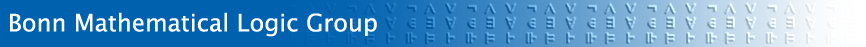## Welcome

to the Mathematical Logic Group at the University of Bonn. Our research areas are set theory and general logic. Please browse our website for details.

### Mathematical logic

Mathematical logic deals with the language of mathematics as well as the mathematics of language. Mathematical statements and arguments can be formulated in formal languages and calculi; formal languages possess mathematical properties. Traditionally, mathematical logic is subdivided into proof theory, model theory, set theory, and computability theory. more ...

### Research

The research of the mathematical logic group is centered around the following topics:

• Set Theory: models of set theory, combinatorics
• Generalized computability theory: ordinal computability
• General Logic: the language of mathematics
• Applications

### Teaching

We teach mathematical logic and set theory in the Bachelor, Master and PhD programs in Mathematics more ...; courses are also open to students of other subjects.

Information on current lectures courses and seminars is available here.

### Contact Information

Office hours Prof. Dr. Peter Koepke by appointment